Definitions of Square Dance Calls and Concepts
Reshape (The Triangle) [C2]

Index -->  Plus  |  A1  |  A2  |  C1  |  C2  |  C3A  |  C3B  |  C4  |  NOL  |
Definitions (Text Only) -->  Plus  |  A1  |  A2  |  C1  |  C2  |  C3A  |  C3B  |  C4  |  NOL  |
 Find call:

 \$B8@8l(B

From a Wave-Based Triangle or Tandem-Based Triangle.

Move forward to the next Triangle position, changing your facing direction (by turning toward the Center of the Triangle) 90° or 180° as appropriate to change a Tandem-Based Triangle into a Wave-Based Triangle or vice-versa.

Wave-Based Triangles \$B\$+\$i(B:

R-H Triangle \$B\$+\$i(B: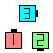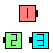Reshape\$B\$NA0(B \$B8e(B
L-H Triangle \$B\$+\$i(B: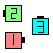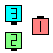Reshape\$B\$NA0(B \$B8e(B

Tandem-Based Triangles \$B\$+\$i(B:

R-H Triangle \$B\$+\$i(B: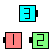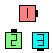Reshape\$B\$NA0(B \$B8e(B
L-H Triangle \$B\$+\$i(B: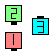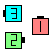Reshape\$B\$NA0(B \$B8e(B

\$BCm
• Reshape The Triangle feels a lot like a Split Counter Rotate 1/4 working to Triangle footprints. In practice, you can dance Reshape as Triangle Counter Rotate 1/4 to footprints, and one of the dancers Roll in order to form a Triangle of the other type. From a Wave-Based Triangle, Reshape feels like Triangle Counter Rotate 1/4, original Apex dancer Roll. From a Tandem-Based Triangle, Reshape feels like Triangle Counter Rotate 1/4, new Apex dancer Roll.
• If you start in a Tandem-Based Triangle, you must end in a Wave-Based Triangle and vice-versa.
• From normal (not Facing or Funny) Triangles, the facing direction of the Apex position does not change.
• Maintain the handedness of the Triangle. If you start in a R-H Triangle you must end in a R-H Triangle.
• 3 By 1 Triangle Reshape [C2]: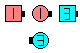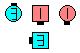3 By 1 Triangle Reshape\$B\$NA0(B \$B8e(BChoreography for Reshape (The Triangle)Comments? Questions? Suggestions?

https://www.ceder.net/def/reshape.php?language=japan
04-April-2020 15:42:36